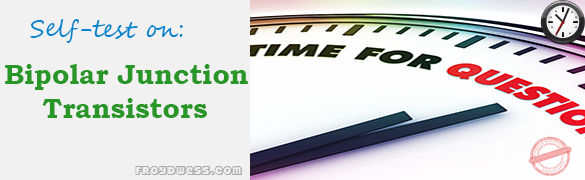# Floyd Self-test in Bipolar Junction Transistors

(Last Updated On: December 8, 2017)This is the Self-test in Chapter 4: Bipolar Junction Transistors from the book Electronic Devices Conventional Current Version, 9th edition by Thomas L. Floyd. If you are looking for a reviewer in Electronics Engineering this will definitely help you before taking the Board Exam.

### Floyd Self-test Chapter 4 Topic Outline

• Floyd Self-test in Bipolar Junction Transistor (BJT) Structure
• Floyd Self-test in Basic BJT Operation
• Floyd Self-test in BJT Characteristics and Parameters
• Floyd Self-test in The BJT as an Amplifier
• Floyd Self-test in The Phototransistor
• Floyd Self-test in The BJT as a Switch
• Floyd Self-test in Transistor Categories and Packaging

### Start Practice Exam Test Questions

Choose the letter of the best answer in each questions.

1. The three terminals of a bipolar junction transistor are called

• (a) p, n, p
• (b) n, p, n
• (c) input, output, ground
• (d) base, emitter, collector

2. In a pnp transistor, the p region are

• (a) base and emitter
• (b) base and collector
• (c) emitter and collector

3. For operation as an amplifier, the base of an npn transistor must be

• (a) positive with respect to the emitter
• (b) positive with respect to the collector
• (c) negative with respect to the emitter
• (d) 0V

4. The emitter current is always

• (a) greater than the base current
• (b) less than the collector current
• (c) greater than the collector current
• (d) answers (a) and (c)

5. The βdc of a transistor is its

• (a) current gain
• (b) voltage gain
• (c) power gain
• (d) internal resistance

6. If Ic is 50 times larger than Ib, then βdc is

• (a) 0.02
• (b) 100
• (c) 50
• (d) 500

7. The approximate voltage across the forward-biased base-emitter junction of silicon BJT is

• (a) 0V
• (b) 0.7
• (c) 0.3
• (d) VBB

8. The bias condition for a transistor to be used as a linear amplifier is called

• (a) forward-reverse
• (b) forward-forward
• (c) reverse-reverse
• (d) collector bias

9. If the output of a transistor amplifier is 5V rms and the input is 100mV rms, the voltage gain is

• (a) 5
• (b) 500
• (c) 50
• (d) 100

10. When a lowercase r’ is used in relation to a transistor, it refers to

• (a) a low resistance
• (b) a wire resistance
• (c) an internal ac resistance
• (d) a source resistance

11. In a given transistor amplifier, Rc= 2.2 kΩ and r’e =20Ω, the voltage gain is

• (a) 2.2
• (b) 110
• (c) 20
• (d) 44

12. When operated in cutoff and saturation, the transistor acts like a

• (a) linear amplifier
• (b) switch
• (c) variable capacitor
• (d) variable resistor

13. In cutoff, Vce is

• (a) 0V
• (b) minimum
• (c) maximum
• (d) equal to Vcc
• (e) answers (a) and (b)
• (f) answers (c) and (d)

14. In saturation, Vce is

• (a) 0.7V
• (b) equal to Vcc
• (c) minimum
• (d) maximum

15. To saturate a BJT,

• (a) Ib= Ic(sat)
• (b) Ib>Ic(sat)/βdc
• (c) Vcc must be at least 10V
• (d) the emitter must be grounded

16. Once in saturation, a further increase in base current will

• (a) cause the collector current to increase
• (b) not affect the collector current
• (c) cause the collector current to decrease
• (d) turn the transistor off

17. In a phototransistor, base current is

• (a) set by a bias voltage
• (b) directly proportional to light intensity
• (c) inversely proportional to light intensity
• (d) not a factor

18. The relationship between the collector current and a light-generated base current is

• (a) Ic= βdcIλ
• (b) Ic= αdcIλ
• (c) Ic= λλ
• (d) Ic= β2dcIλ

19. An optocoupler usually consists of

• (a) two LEDs
• (b) an LED and a photodiode
• (c) an LED and a phototransistor
• (d) both (b) and (c)

20. In a transistor amplifier, if the base-emitter junction is open, the collector voltage is

• (a) Vcc
• (b) 0V
• (c) floating
• (d) 0.2V

21. A DMM measuring on open transistor junction shows

• (a) 0V
• (b) 0.7V
• (c) OL
• (d) Vcc

### Check your work.

Floyd Self-test in Bipolar Junction Transistors
4 (80%) 1 vote